Start Now with 100 Free Questions

# CAT4 – Spatial Reasoning

## What includes in the CAT4 Spatial Reasoning?

The CAT4 Spatial Reasoning section is composed of several subtests that assess a student’s ability to think and reason about visual and spatial relationships. The specific subtests included in the Spatial Reasoning section may vary depending on the level of the test and the testing organization, but generally, figure analysis and figure recognition are included. These subtests, along with examples, are explained below.

## Figure Analysis

This subtest presents the student with a square that is folded once or more and then has one or more holes punched into it. Students must work out what the paper will look like when it is unfolded and select the answer from choices given.  This assesses a student’s ability to mentally create a complex visual image, retain it, and manipulate it before comparing the imagined result with the answer choices given.

Examples of Figure Analysis questions increase from lower-level questions to higher-level questions.

Instruction:  Take a look at the pictures across the top.  They show a piece of square paper being folded.  Then, one or more holes are punched in the folded piece of paper.  Which answer shows what the square piece of paper will look like when it is unfolded?

Example 1: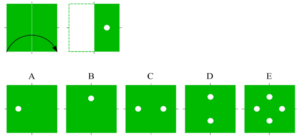Answer:  C – The square piece of paper is folded in half vertically. A hole is punched in the middle of the folded sheet. When unfolded, the square piece of paper now has two holes.

Example 2: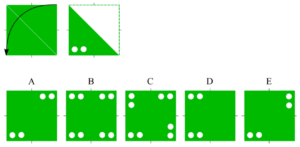Answer:  E – The square piece of paper is folded in half diagonally. Two holes are punched in the lower left of the folded sheet. When unfolded, the square piece of paper now has four holes; two in the lower left corner along the horizontal edge and two in the upper right corner along the vertical edge.

Example 3: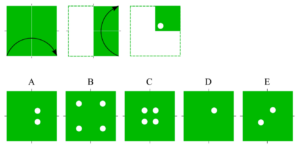Answer:  E – The square piece of paper is folded vertically from left to right and then folded up horizontally once more to make a smaller square piece of paper. A hole is punched in the middle left corner of the folded sheet. When unfolded, the square piece of paper now has four holes in the middle of the paper.

Example 4: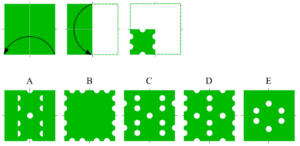Answer:  C – The square piece of paper is folded in half vertically from right to left and then folded down horizontally once more to make a smaller square piece of paper. Six holes are punched in the smaller square sheet of paper; two on the left edge, two on the right edge, one on the top, and one on the bottom. When the paper is unfolded, it now has six complete holes and 12 half-punched holes along the edges of the paper.

## Figure Recognition

This subtest presents the student with five complex figures and a target figure.  Students must identify which of the five figures contains the exact same size partial piece of the target.  This subtest assesses visualization skills, especially the ability to retain mental images of a figure that represents angles and lengths accurately.

Instruction:  A single shape is given. Find the hidden shape in the answer choices provided.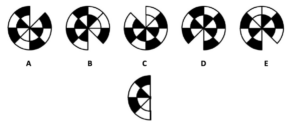Answer:  A – The target is the same as the left half of the answer choice.

Overall, the Spatial Reasoning section of the CAT4 is designed to assess a student’s ability to think and reason about visual and spatial relationships. The questions in this section may involve recognizing and matching two-dimensional figures based on their visual and spatial characteristics and visualizing and mentally manipulating a two-dimensional shape as it is folded and unfolded.  Nonverbal Reasoning and Spatial Ability tests can indicate a real aptitude for subjects such as mathematics, physics, design, engineering, architecture, and other fields which draw on spatial abilities.